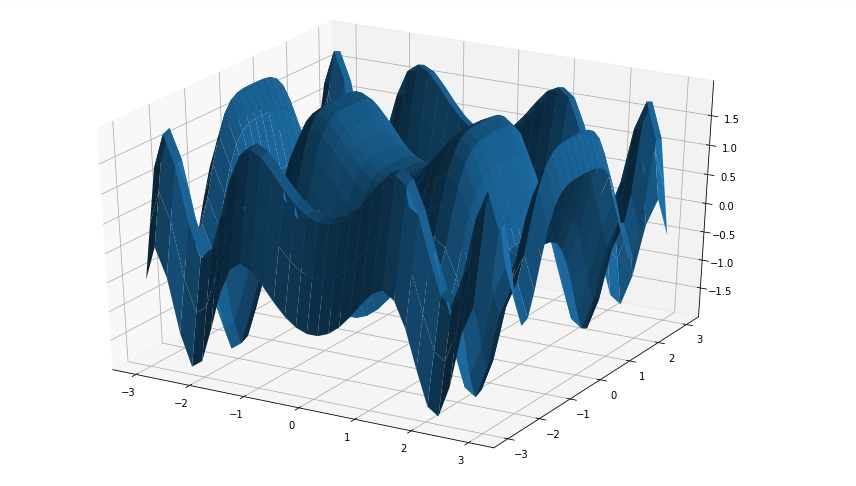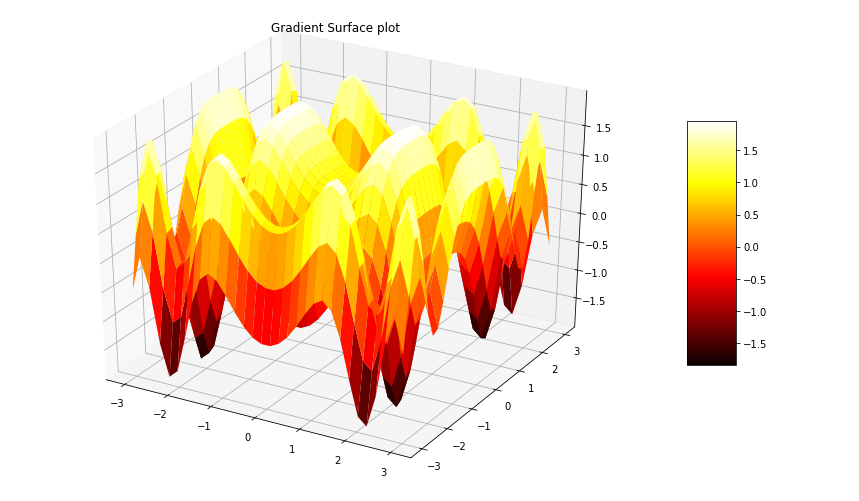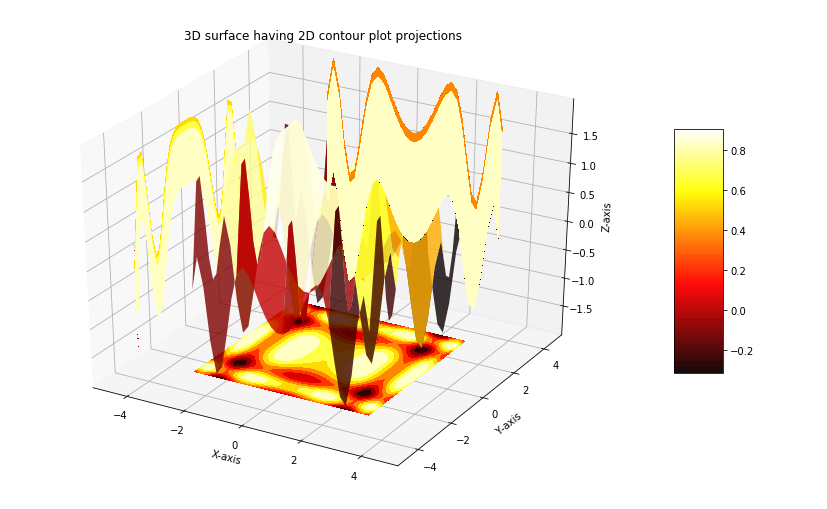# 3D Surface plotting in Python using Matplotlib

A Surface Plot is a representation of three-dimensional dataset. It describes a functional relationship between two independent variables X and Z and a designated dependent variable Y, rather than showing the individual data points. It is a companion plot of the contour plot. It is similar to the wireframe plot, but each face of the wireframe is a filled polygon. This helps to create the topology of the surface which is being visualized.

## Creating 3D surface Plot

The `axes3d` present in Matplotlib’s `mpl_toolkits.mplot3d` toolkit provides the necessary functions used to create 3D surface plots.Surface plots are created by using `ax.plot_surface()` function.

Syntax:

`ax.plot_surface(X, Y, Z)`

where X and Y are 2D array of points of x and y while Z is 2D array of heights.Some more attributes of `ax.plot_surface()` function are listed below:

Attribute Description
X, Y, Z 2D arrays of data values
cstride array of column stride(step size)
rstride array of row stride(step size)
ccount number of colums to be used, default is 50
rcount number of row to be used, default is 50
color color of the surface
cmap colormap for the surface
norm instance to normalize values of color map
vmin minimum value of map
vmax maximum value of map
facecolors face color of individual surface

Example: Let’s create a 3D surface by using the above function

 `# Import libraries ` `from` `mpl_toolkits ``import` `mplot3d ` `import` `numpy as np ` `import` `matplotlib.pyplot as plt ` ` `  ` `  `# Creating dataset ` `x ``=` `np.outer(np.linspace(``-``3``, ``3``, ``32``), np.ones(``32``)) ` `y ``=` `x.copy().T ``# transpose ` `z ``=` `(np.sin(x ``*``*``2``) ``+` `np.cos(y ``*``*``2``) ) ` ` `  `# Creating figyre ` `fig ``=` `plt.figure(figsize ``=``(``14``, ``9``)) ` `ax ``=` `plt.axes(projection ``=``'3d'``) ` ` `  `# Creating plot ` `ax.plot_surface(x, y, z) ` ` `  `# show plot ` `plt.show() `

Output:Gradient surface plot is a combination of 3D surface plot with a 2D contour plot. In this plot the 3D surface is colored like 2D contour plot. The parts which are high on the surface contains different color than the parts which are low at the surface.

Syntax:

surf = ax.plot_surface(X, Y, Z, cmap=, linewidth=0, antialiased=False)

The attribute `cmap=` stes the color of the surface. A color bar can also be added by calling `fig.colorbar`. The code below create a gradient surface plot:

Example:

 `# Import libraries ` `from` `mpl_toolkits ``import` `mplot3d ` `import` `numpy as np ` `import` `matplotlib.pyplot as plt ` ` `  `# Creating dataset ` `x ``=` `np.outer(np.linspace(``-``3``, ``3``, ``32``), np.ones(``32``)) ` `y ``=` `x.copy().T ``# transpose ` `z ``=` `(np.sin(x ``*``*``2``) ``+` `np.cos(y ``*``*``2``) ) ` ` `  `# Creating figyre ` `fig ``=` `plt.figure(figsize ``=``(``14``, ``9``)) ` `ax ``=` `plt.axes(projection ``=``'3d'``) ` ` `  `# Creating color map ` `my_cmap ``=` `plt.get_cmap(``'hot'``) ` ` `  `# Creating plot ` `surf ``=` `ax.plot_surface(x, y, z, ` `                       ``cmap ``=` `my_cmap, ` `                       ``edgecolor ``=``'none'``) ` ` `  `fig.colorbar(surf, ax ``=` `ax, ` `             ``shrink ``=` `0.5``, aspect ``=` `5``) ` ` `  `ax.set_title(``'Surface plot'``) ` ` `  `# show plot ` `plt.show() `

Output:## 3D surface Plot having 2D contour plot projections

3D surface plots plotted with Matplotlib can be projected on 2D surfaces. The code below creates a 3D plots and visualizes its projection on 2D contour plot:

Example:

 `# Import libraries ` `from` `mpl_toolkits ``import` `mplot3d ` `import` `numpy as np ` `import` `matplotlib.pyplot as plt ` ` `  `# Creating dataset ` `x ``=` `np.outer(np.linspace(``-``3``, ``3``, ``32``), np.ones(``32``)) ` `y ``=` `x.copy().T ``# transpose ` `z ``=` `(np.sin(x ``*``*``2``) ``+` `np.cos(y ``*``*``2``) ) ` ` `  `# Creating figyre ` `fig ``=` `plt.figure(figsize ``=``(``14``, ``9``)) ` `ax ``=` `plt.axes(projection ``=``'3d'``) ` ` `  `# Creating color map ` `my_cmap ``=` `plt.get_cmap(``'hot'``) ` ` `  `# Creating plot ` `surf ``=` `ax.plot_surface(x, y, z,  ` `                       ``rstride ``=` `8``, ` `                       ``cstride ``=` `8``, ` `                       ``alpha ``=` `0.8``, ` `                       ``cmap ``=` `my_cmap) ` `cset ``=` `ax.contourf(x, y, z, ` `                   ``zdir ``=``'z'``, ` `                   ``offset ``=` `np.``min``(z), ` `                   ``cmap ``=` `my_cmap) ` `cset ``=` `ax.contourf(x, y, z, ` `                   ``zdir ``=``'x'``, ` `                   ``offset ``=``-``5``, ` `                   ``cmap ``=` `my_cmap) ` `cset ``=` `ax.contourf(x, y, z,  ` `                   ``zdir ``=``'y'``, ` `                   ``offset ``=` `5``, ` `                   ``cmap ``=` `my_cmap) ` `fig.colorbar(surf, ax ``=` `ax,  ` `             ``shrink ``=` `0.5``, ` `             ``aspect ``=` `5``) ` ` `  `# Adding labels ` `ax.set_xlabel(``'X-axis'``) ` `ax.set_xlim(``-``5``, ``5``) ` `ax.set_ylabel(``'Y-axis'``) ` `ax.set_ylim(``-``5``, ``5``) ` `ax.set_zlabel(``'Z-axis'``) ` `ax.set_zlim(np.``min``(z), np.``max``(z)) ` `ax.set_title(``'3D surface having 2D contour plot projections'``) ` ` `  `# show plot ` `plt.show() `

Output:My Personal Notes arrow_drop_upCheck out this Author's contributed articles.

If you like GeeksforGeeks and would like to contribute, you can also write an article using contribute.geeksforgeeks.org or mail your article to contribute@geeksforgeeks.org. See your article appearing on the GeeksforGeeks main page and help other Geeks.

Please Improve this article if you find anything incorrect by clicking on the "Improve Article" button below.

Article Tags :

Be the First to upvote.

Please write to us at contribute@geeksforgeeks.org to report any issue with the above content.